# First variation formula

## Statement

### Notation

Let$M$ be a Riemannian manifold and$p,q \in M$. Let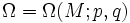$\Omega = \Omega(M;p,q)$ be the path space of$M$, viz the space of piecewise smooth paths from$p$ to$q$.

Let the points of non-smoothness by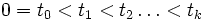$0=t_0 < t_1 < t_2 \ldots < t_k$. Let$\alpha$ be a variation of$\omega$, and$W$ be its variation vector field. Let$V_t = d\omega/dt$ wherever$\omega$ is differentiable. For each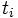$t_i$ where$\omega$ is not smooth, let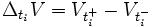$\Delta_{t_i}V = V_{t_i^+} - V_{t_i^-}$.

Denote by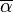$\overline{\alpha}$ the map sending$u$ to the curve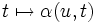$t \mapsto \alpha(u,t)$.

### The formula$\frac{1}{2} \frac{dE(\overline{\alpha}(u))}{du} = - \sum_{i=1}^{k-1} - \int_0^1 dt$